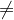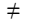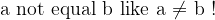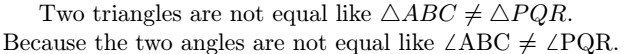# How to write not equal symbol in LaTeX like a ≠ b?

You can easily define the equal symbol in latex because this symbol is on your computer keyboard.

However, in the case of not equal symbol, you need to use different commands or packages.

Symbol Not equal symbol
Type of symbol Inequality
Package MnSymbol, stix, …, etc
Argument No
Latex command \neq,\ne
Example X \neq Y → X ≠ Y

Take a look at this table below for your understanding, where the command, as well as the package, are simply mentioned.

 \neq \ne [defult]\nequal \neq \ne [MnSymbol]\nequal \neq \ne [fdsymbol]\neq \ne [boisk]\neq \ne [stix]It is enough to know the use of a single command to represent the not equal symbol in latex.

But, even after that, each method has been discussed in-depth in this tutorial to further enhance your knowledge. So, let’s learn deeply …..

## Use default command in LaTeX

Default command means you do not have to use any external package for this.

Already, particular commands have been defined for particular symbols in latex.

\documentclass{article}
\begin{document}
$$a \ne b$$
$$p \neq q$$
$$p \neq q \ne r$$
$$\triangle ABC \neq \triangle PQR$$
\end{document}

Output :## Use MnSymbol and fdsymbol packages

Both MnSymbol and fdsymbol packages have the same command present. In this case, the shape of the symbol is somewhat different than before.

\documentclass{article}
\usepackage{MnSymbol,fdsymbol}
\begin{document}
$$a \nequal b$$
$$p \nequal q$$
$$p \ne q \neq r$$
\end{document}

Output :## Use \not command before an equal symbol

If you use the \not command before the equal symbol, it will return you not equal symbol.

Of course, this is an interesting method of representing the not equal symbol.

\documentclass{article}
\begin{document}
$$a \not = b$$
$$p \not = q \not = r$$
$$\triangle ABC \not = \triangle PQR$$
\end{document}

Output :And you can create new commands without using \not = syntax direct, which will look more cool for you.

\documentclass{article}
\newcommand{\notequal}{$\not =$ }
\begin{document}
a not equal to b like a \notequal b !
\end{document}

Output :## A not equal symbol in text mode

There is no direct command to represent the not equal symbol in the text. You can import the not equal symbol in the text using a single dollar on both sides of each command.

\documentclass{article}
\newcommand{\notequal}{$\not =$ }
\begin{document}
Two triangles are not equal like $\triangle ABC \neq \triangle PQR$. \\
Because the two angles are not equal like \angle ABC \notequal \angle PQR.
\end{document}

Output :## Use not equal symbol with other symbols

You will notice that in different mathematical books, not equal symbol is used with different inequality symbols to represent an expression. Such as greater than, less than, and subset, etc.

\documentclass{article}
\usepackage{fdsymbol,mathabx,amsmath}
\begin{document}
$$\precneqq \;\;\; \succneqq$$
$$\subsetneqq \;\;\; \supsetneqq$$
$$\sqsubsetneqq \;\;\; \sqsupsetneqq$$
$$\lneqq \;\;\; \gneqq$$
\end{document}

Output :## Not equal operator in LaTeX

Not equal operator is comparison operators used in programming languages. And in this case != and !== symbols are used instead of ≠ symbols.

\documentclass{article}
\begin{document}
$$a != b$$
$$p != q$$
$$a !== b$$
\end{document}

Output :#### Md Jidan Mondal

LaTeX expert with over 10 years of experience in document preparation and typesetting. Specializes in creating professional documents, reports, and presentations using LaTeX.